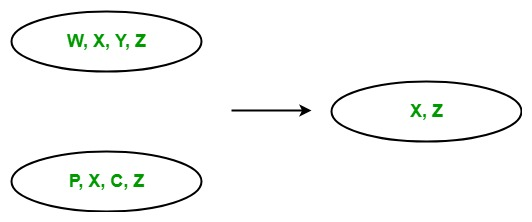# LINQ | Set Operator | Intersect

• Last Updated : 22 May, 2019

In LINQ, Set operators are those operators in query expression which return a result set which is based on the existence or non-existence of the equivalent elements within the same or different collections or sequences or sets. The standard query operator contains the following set operators:

1. Union
2. Intersect
3. Except
4. Distinct

#### Intersect Operator

The Intersect operator returns the set intersection. Or in other words, we can say that it return the set or collection which contain the common elements appears in two collections, or sets.• It does not support query syntax in C# and VB.Net languages. But you can use Intersect method on query variable or you can wrap your query in brackets and then the call Intersect method.
• It support method syntax in both C# and VB.Net languages.
• It present in both the Queryable and Enumerable class.
• It is implemented by using deferred execution.
• When you are working with the collections of complex types, then you must use IEqualityComparer interface, otherwise the Intersect method will give you incorrect result.

Example 1:

 `// C# program to find the intersection``// of the given sequence``using` `System;``using` `System.Linq;`` ` `class` `GFG {`` ` `    ``static` `public` `void` `Main()``    ``{`` ` `        ``// Data source``        ``char``[] sequence1 = {``'m'``, ``'q'``, ``'o'``,``                           ``'s'``, ``'y'``, ``'a'``};`` ` `        ``char``[] sequence2 = {``'p'``, ``'t'``, ``'r'``, ``                           ``'s'``, ``'y'``, ``'z'``};`` ` `        ``// Display the sequences``        ``Console.WriteLine(``"Sequence 1 is: "``);`` ` `        ``foreach``(``var` `s1 ``in` `sequence1)``        ``{``            ``Console.WriteLine(s1);``        ``}`` ` `        ``Console.WriteLine(``"Sequence 2 is: "``);`` ` `        ``foreach``(``var` `s2 ``in` `sequence2)``        ``{``            ``Console.WriteLine(s2);``        ``}`` ` `        ``// Get intersection of the given sequence``        ``// Using Intersect function``        ``var` `result = sequence1.Intersect(sequence2);`` ` `        ``Console.WriteLine(``"New Sequence: "``);`` ` `        ``foreach``(``var` `val ``in` `result)``        ``{``            ``Console.WriteLine(val);``        ``}``    ``}``}`
Output:
```Sequence 1 is:
m
q
o
s
y
a
Sequence 2 is:
p
t
r
s
y
z
New Sequence:
s
y
```

Example 2:

 `// C# program to find the commonly``// known languages by the employees``using` `System;``using` `System.Linq;``using` `System.Collections.Generic;`` ` `// Employee details``public` `class` `Employee1 {`` ` `    ``public` `int` `emp_id1``    ``{``        ``get``;``        ``set``;``    ``}`` ` `    ``public` `string` `emp_name1``    ``{``        ``get``;``        ``set``;``    ``}``    ``public` `string` `emp_lang1``    ``{``        ``get``;``        ``set``;``    ``}``}`` ` `// Employee details``public` `class` `Employee2 {`` ` `    ``public` `int` `emp_id2``    ``{``        ``get``;``        ``set``;``    ``}`` ` `    ``public` `string` `emp_name2``    ``{``        ``get``;``        ``set``;``    ``}``    ``public` `string` `emp_lang2``    ``{``        ``get``;``        ``set``;``    ``}``}`` ` `public` `class` `GFG {`` ` `    ``// Main method``    ``static` `public` `void` `Main()``    ``{``        ``List emp1 = ``new` `List() {`` ` `            ``new` `Employee1() {emp_id1 = 209, emp_name1 = ``"Anjita"``,``                                               ``emp_lang1 = ``"C#"``},`` ` `            ``new` `Employee1() {emp_id1 = 210, emp_name1 = ``"Soniya"``,``                                                ``emp_lang1 = ``"C"``},`` ` `            ``new` `Employee1() {emp_id1 = 211, emp_name1 = ``"Rohit"``,``                                            ``emp_lang1 = ``"Java"``},`` ` `        ``};`` ` `        ``List emp2 = ``new` `List() {`` ` `            ``new` `Employee2() {emp_id2 = 290, emp_name2 = ``"Anjita"``,``                                               ``emp_lang2 = ``"C#"``},`` ` `            ``new` `Employee2() {emp_id2 = 212, emp_name2 = ``"MaMa"``,``                                         ``emp_lang2 = ``"Python"``},`` ` `            ``new` `Employee2() {emp_id2 = 233, emp_name2 = ``"Rima"``,``                                           ``emp_lang2 = ``"Java"``},`` ` `        ``};`` ` `        ``// Query to find the commonly known``        ``// languages by the employees``        ``// Using Intersect method``        ``var` `res = emp1.Select(e => e.emp_lang1).Intersect(emp2.Select(e => e.emp_lang2));``         ` `        ``Console.WriteLine(``"Commonly known Languages: "``);``         ` `        ``foreach``(``var` `val ``in` `res)``        ``{``            ``Console.WriteLine(val);``        ``}``    ``}``}`
Output:
```Commonly known Languages:
C#
Java
```

My Personal Notes arrow_drop_up